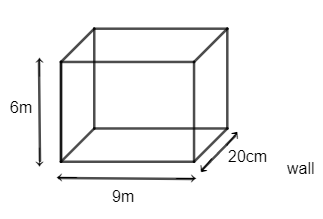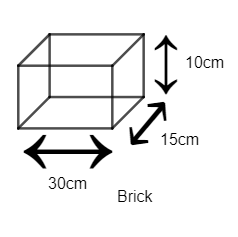QuestionAnswers

# State whether true or false.A wall 9 m long, 6 m high and 20 cm thick, is to be constructed using bricks of dimensions 30 cm, 15 cm and 10 cm. The number of bricks required will be 2400.A.TrueB.FalseHint: Volume represents the capacity of any solid. Volume of any cuboid with length as ‘l’, breadth as ‘b’ and height as ‘h’ can be given as $=l\times b\times h$. Get volumes of brick and wall using the given dimensions. Calculate the total volume of the wall using the volume of brick and number of the wall using the wall using the volume of brick and number of bricks involved to construct the wall.
Now, equate them to get the value of bricks as both represent the same volume of a solid.

We know that wall brick will be of the cuboid shape and the wall is constructed using the bricks. It means the volume of the total number of bricks involved to construct the wall should be equal to the volume of the wall because volume represents capacity of any solid within itself.
Let there be ‘n’ bricks involved to construct the wall of a given dimension.
So, we know the volume of any cuboid with given measurement as
Volume of cuboid $=length\times breadth\times height$ ……………………………….(i)
Now, we have dimension of wall as
Length $=9m$
Height $=6m$
Breadth (thickness) $=20cm$Now, we know
$1m=100cm$
Hence, length of the wall in cm can be given as
Length $9m=9\times 100cm=900cm$
Similarly, height can be given as
$6m=6\times 100cm=600cm$
Volume of the wall can be given as
Volume of the wall $=900\times 600\times 20c{{m}^{3}}$ ………………………..(ii)
Now, we can get volume for brick using equation (i)
So, dimensions of a brick are given as $\left( 30cm,15cm,10cm \right)$.Hence, volume of a brick can be calculated as
Volume of a brick $=30\times 15\times 10c{{m}^{3}}$ …..................................(iii)
Now, we have already supposed that ‘n’ bricks are involved for making the wall of given dimensions.
So, the volume of n bricks can be calculated by multiplying ‘n ‘ by the volume of one brick from the equation (iii). Hence, we get
Volume of n bricks $=n\times 30\times 15\times 10c{{m}^{3}}$ …………………………………..(iv)
Now, we know that ’n’ bricks are involved to make the wall, hence volume of both of them should be equal as n bricks are occupying the spaces of the wall.
Hence, from equation(ii) and (iv), we get
$900\times 600\times 20=n\times 30\times 15\times 10$
$n=\dfrac{900\times 600\times 20}{30\times 15\times 10}$
$n=30\times 40\times 2$
$n=2400$
Hence, the given statement is true that the number of bricks required to make a wall of given dimensions by a brick with the provided dimension are 2400.$=n=2400$
Hence, option (A) is correct.

Note: Equating volume of both the rectangular cuboids (bricks and wall) is the key point of the question. One may get confused and he/she tries to equate the surface areas of both of them which is the wrong approach as the surface area of the bricks and wall are not equal as Area does not represent the capacity of any solid.
Conversion of units of any dimension is the key point of the question as well. Try to use ‘cm’ or ‘m’ for representing the dimension, i.e. use only one unit for these kinds of questions.
View Notes
CBSE Class 6 Maths Chapter 10 - Mensuration FormulasCBSE Class 9 Maths Chapter 13 - Surface Areas and Volumes FormulasCBSE Class 10 Maths Chapter 13 - Surface Areas and Volumes Formula2D and 3D FiguresGram-Negative Bacteria Cell WallCBSE Class 10 Maths Chapter 6 - Triangles FormulaSurface Area of a Prism FormulaCBSE Class 9 Maths Chapter 6 - Lines and Angles FormulasCBSE Class 10 Maths Chapter 10 - Circles FormulaCBSE Class 8 Maths Chapter 11 - Mensuration FormulasCBSE Class 10 Hindi A Question Paper 2020Hindi A Class 10 CBSE Question Paper 2009Hindi A Class 10 CBSE Question Paper 2015Hindi A Class 10 CBSE Question Paper 2016Hindi A Class 10 CBSE Question Paper 2012Hindi A Class 10 CBSE Question Paper 2010Hindi A Class 10 CBSE Question Paper 2007Hindi A Class 10 CBSE Question Paper 2013Hindi A Class 10 CBSE Question Paper 2008Hindi A Class 10 CBSE Question Paper 2014NCERT Solutions for Class 6 Maths Chapter 10RD Sharma Solutions for Class 9 Maths Chapter 21 - Surface Area and Volume of a SphereRD Sharma Solutions for Class 9 Maths Chapter 18 - Surface Area and Volume of a Cuboid and CubeRD Sharma Class 10 Solutions Chapter 9 - Exercise 9.6RD Sharma Class 9 Solutions Chapter 10 - Congruent Triangles (Ex 10.6) Exercise 10.6RD Sharma Solutions for Class 9 Maths Chapter 19 - Surface Area and Volume of A Right Circular CylinderRD Sharma Solutions for Class 9 Maths Chapter 20 - Surface Area and Volume of A Right Circular ConeNCERT Solutions for Class 9 Maths Chapter 10 Circles (Ex 10.6) Exercise 10.6RD Sharma Class 6 Solutions Chapter 6 - Fractions (Ex 6.9) Exercise 6.9NCERT Solutions for Class 10 Maths Chapter 6 Triangles (Ex 6.6) Exercise 6.6